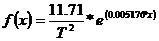# Team:OUC-China/Magnetic Analysis

Model in RNA guardian

### Abstract

We built a model to qualitatively and quantitatively analyze the magnetic detection ability of the sample bacteria, introducing a magnetism detection coefficient to quantify this ability. We were successful in using random function to simulate the movement process inside the microfluidic chip, thus comparing the modeling results with the experimental results, eliminating systematic error. The experimental results showed that although the membrane-producing gene cluster was inserted into the E.coli cells, it did not endow the cell with evident magnetic detection ability.

### Introduction

Because the distribution of the Magnetospirillum Magneticum AMB-1 and engineered bacteria in culture is random, it is difficult to detect Magnetospirillum Magneticum  AMB-1 and engineered bacteria’s ability of feeling magnetism directly. We use microfluidic chip to make sure Magnetospirillum Magneticum  AMB-1 and engineered bacteria move in a fixed direction. Then magnetic field in the vertical direction of the fixed direction can make Magnetospirillum Magneticum  AMB-1 and engineered bacteria produce a orthogonal movement. We used mathematical modeling to qualitative and quantitative analyze the distance bacteria travelled in the vertical direction of the fixed direction by detecting distribution density of Magnetospirillum Magneticum AMB-1 and engineered bacteria in magnetic field. In order to quantify Magnetospirillum Magneticum AMB-1 and engineered bacteria’s ability of detecting magnetism ,we introduce a new parameter and manage to figure out the coefficient values .

### Model assumption

Magnetospirillum Magneticum AMB-1 and engineered bacteria’s magnetism detection coefficient are both the same.

### Symbols description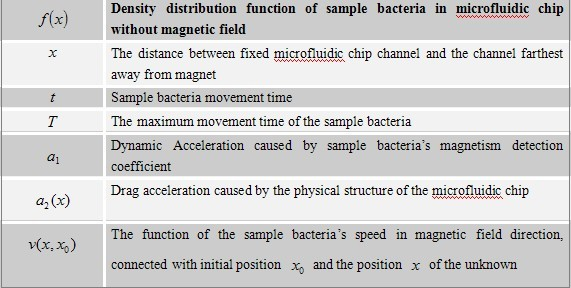### Models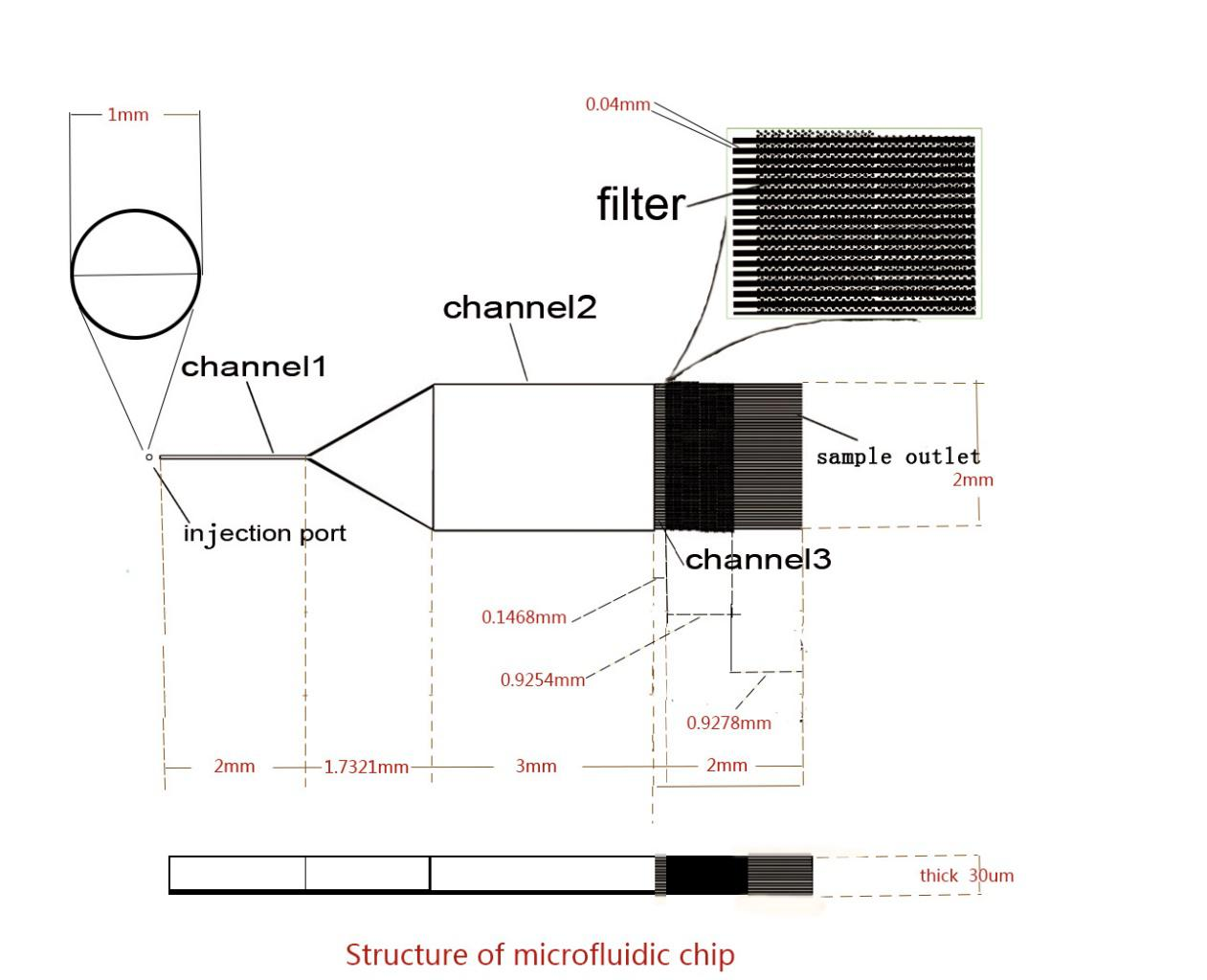Microfluidic chip, as shown

Culture fluid enters into the microfluidic chip from the injection port. After passing though channel 2, sample bacteria broth remains in the filter in channel 3, while culture fluid issues from the sample outlet. The movement of sample bacteria in channel 2 can be divided into two parts, free movement along the channel and offset movement in the direction of the magnetic field.
Ignoring the trail of the sample bacteria’s movement, only considering the sample bacteria’s final position without magnetic field and offset in the magnetic field, we got the following results.
Under no magnetic field conditions, the sample bacteria’s distribution in the microfluidic chip shall meet:
f(x)

When adding the magnetic field, the movement of sample bacteria is affected by the fixed magnetic field, resulting in a force parallel to the magnetic field and a certain dynamic acceleration.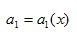These makes the sample bacteria tend to move towards the direction of the magnetic field. The sample bacteria’s movement is restricted by the size of the microfluidic chip, so the closer the bacteria gets to the border of the microfluidic chip, the more resistance the bacteria feels. And also, the drag acceleration resulting from the resistance changes with the bacteria’ position in the microfluidic chip. The drag acceleration meets this equation: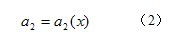The resistance makes the sample bacteria form a certain density distribution, so we can observe the distribution of the bacteria in magnetic-free field and then work out the friction drag and the correspondent drag acceleration. From (1) and (2), we can get the functional relationship between the original position x1 and terminal position x2: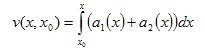Because the speed is the derivative of the distance with respect to time.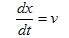And then work out the functional relationship between the distance and time: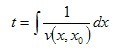And because the sample bacteria’s termination of the offset resulting from the magnetic field meets the following relationship: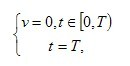We can take x0 into the equation one by one, and then define the corresponding point offset as well as the final sample bacteria’s density distribution function. Furthermore, the final density distribution function only depends on four parameters,x0,T,a1 and a2. As x0, T are certain parameters, a2 is dependant on the sample bacteria’s distribution function under no magnetic field conditions. Therefore, the corresponding point offset only depends on a1, namely the sample bacteria’s magnetism detection coefficient. So we can use the sample bacteria’s distribution function under two conditions, both with a magnetic field and without.

### Model Test

Through the experiment, we get data.
According to the blank control group, we find that the density distribution of sample bacteria is Random distribution.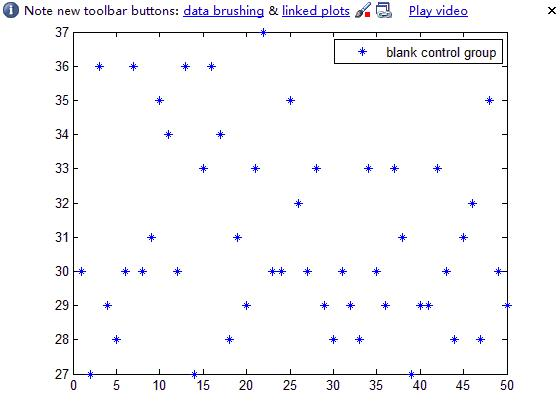Fig.3.1 the distribution of E.coli in microfluidic chip without magnetic field

So the distribution function of sample bacteria is random function. We established a stochastic model to simulate the process of the sample bacteria’s movement in microfluidic chip.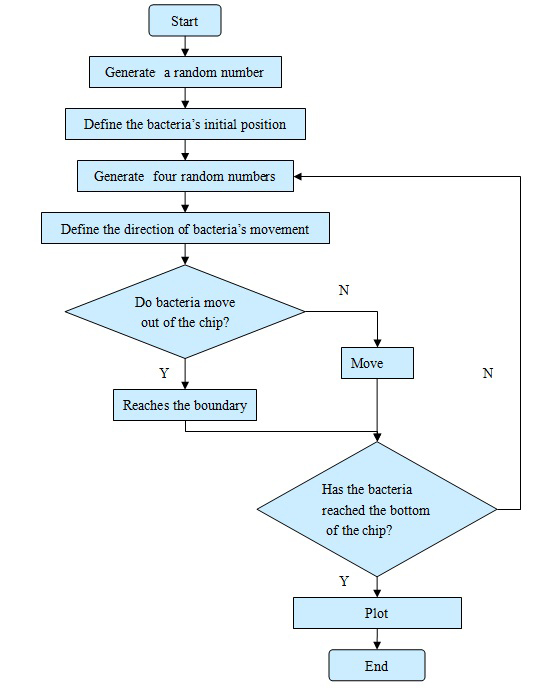Fig.1.1 Simulation experiment loop

We did regression analysis to the simulation experiment results and the practical experiment results separately, and we found that the analysis satisfied the condition as follows: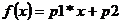We managed to find the distribution center and the circumambient distribution function. Then we compared the differences between different coefficients by calculating the circumambient distribution function. After calculating, the difference between the simulation experiment and the practical experiment is showed as these: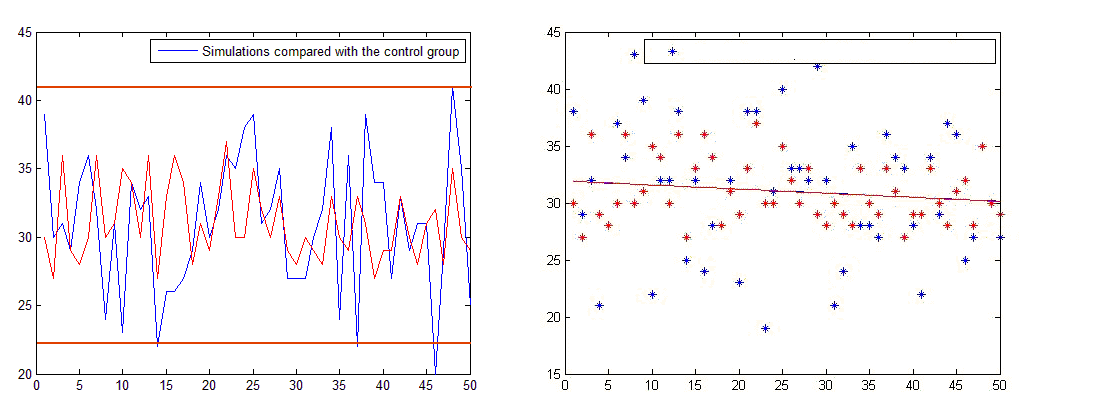Fig.1.2 simulation about engineered bacteria compared with the control group. The red line and the red points show the real data, the blue line and blue points show the simulated data. In the right-hand picture, the blue line has been blocked by the red line, because they coincide almost perfectly.

As we can see, the circumambient distribution function of the simulation experiment and the practical experiment is particularly similar. So we concluded that the simulation experiment’s result is believable. Based on the analysis above, we can use the method applied to the simulation experiment to make an analysis on the experimental group (under the condition that the magnetic field exists). In this way, we can avoid the systematic errors caused by the differences of total numbers of sample bacteria between different experimental groups. We can also see the magnetic field’s influence on the sample bacteria more visually.

Based on the analysis above, we made the same simulation experiment on another two experimental groups to compare the change of the sample distribution, under the condition of magnetic and magnetic-free field.On the magnetic field strength of 205mG: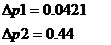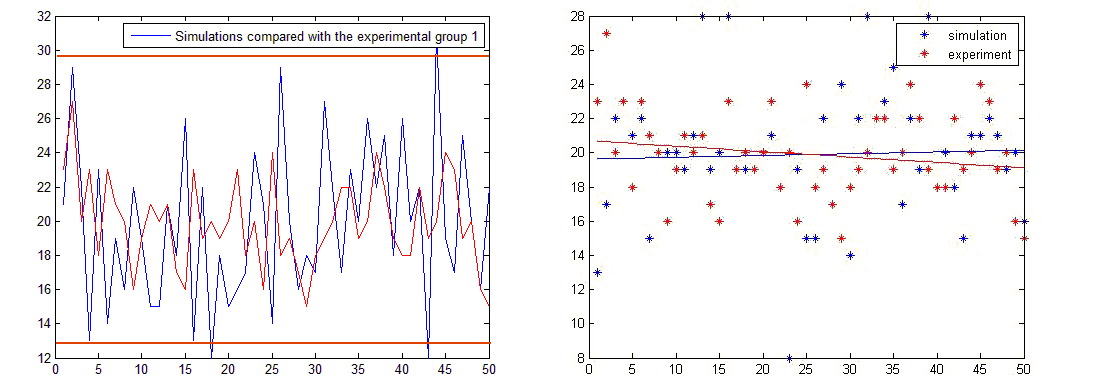Fig.1.3 simulation about engineered bacteria compared with the experimental group 1

Under the magnetic field strength of 250mG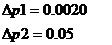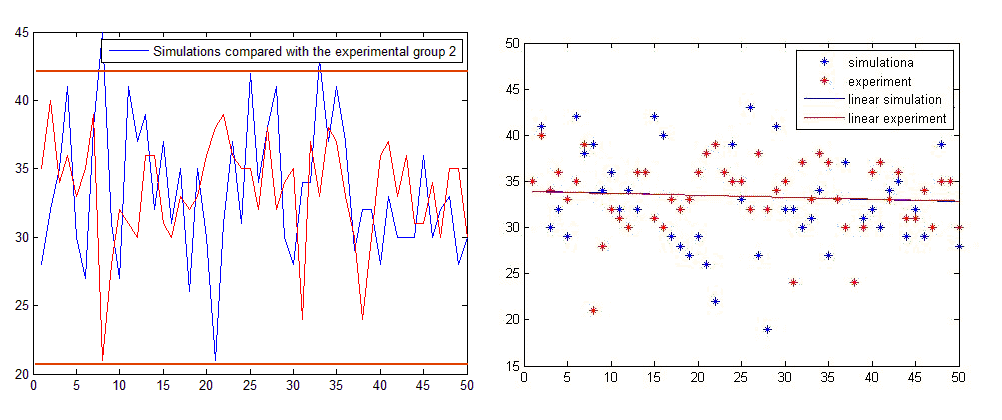Fig.1.4 simulation about engineered bacteria compared with the experimental group 2 . In the right-hand picture, the blue line has been blocked by the red line, because they coincide almost perfectly.

Comparing the two results, we can find that, under the condition of a magnetic field with a strength less than 250mG, we cannot observe the effect of the magnetic field on the engineered bacteria, that is to say, the engineered bacteria can’t sense the magnetic field.

We make a similar simulation on the experimental group and compare the different distributions in a magnetic field or a magnetic-free field. The sample responds strongly to magnetic force and we find that the function of bacteria density in the blank control group is several constants composited with uniform distribution. And we concluded that the drag acceleration is zero.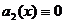We finally calculated that the density distribution of the bacteria in magnetic field is approximately a piecewise linear function.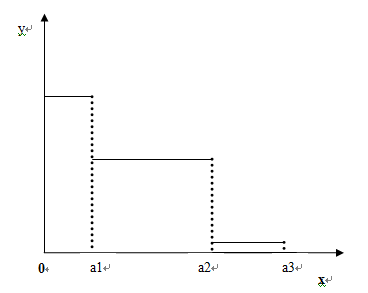Fig.1.5 simulation about Magnetospirillum Magnetism AMB-1 compared with the experimental group1.

We assume that the density distribution of the Magnetospirillum Magnetism AMB-1 can be described with the previous picture. With the function in the models, we can calculate the dynamic acceleration.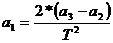Rearranging the dynamic acceleration equation and the function, we finally get the relationship between the dynamic acceleration and the magnetic intensity.Testing the relationship with data:
Experimental group 1 （magnetic field intensit：164.8mG）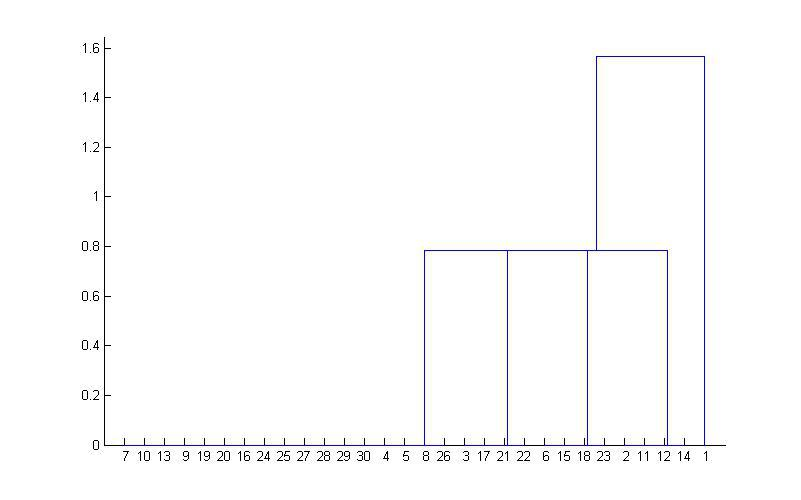Fig.1.6 Clustering results about Magnetospirillum Magnetism AMB-1 experimental group1.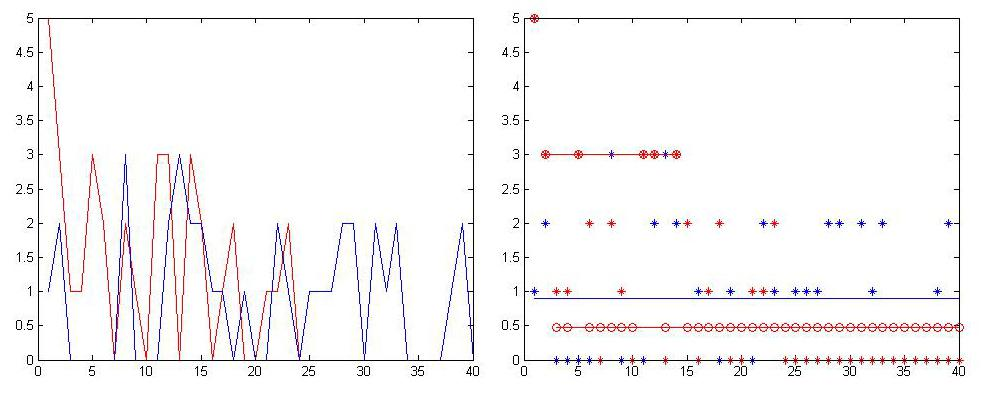Fig.1.7 Simulation about Magnetospirillum Magnetism AMB-1 compared with the experimental group1.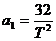Experimental group 2 （magnetic field intensit：212.0mG）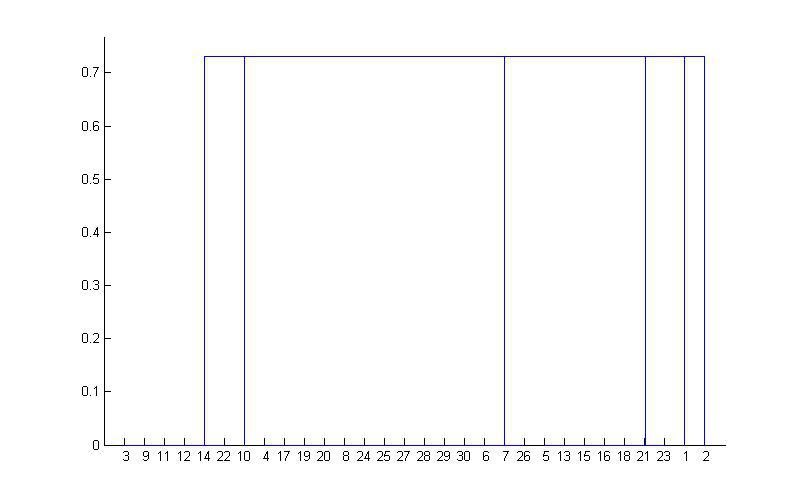Fig.1.8 Clustering results about Magnetospirillum Magnetism AMB-1 experimental group2.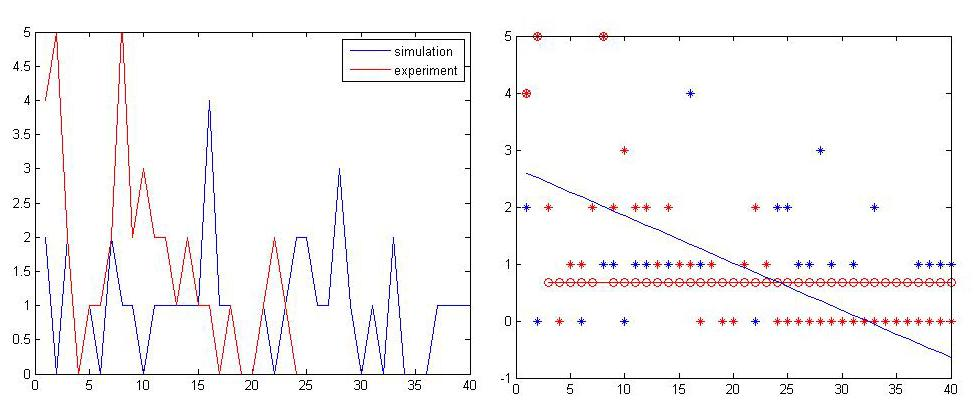Fig.1.9 simulation about Magnetospirillum Magnetism AMB-1 compared with the experimental group2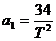Experimental group 3 （magnetic field intensit：230.0mG）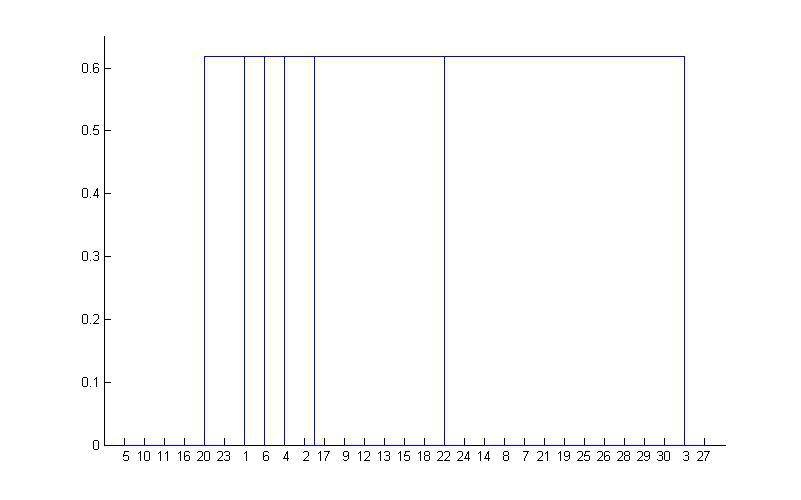Fig.1.10 Clustering results about Magnetospirillum Magnetism AMB-1 experimental group3.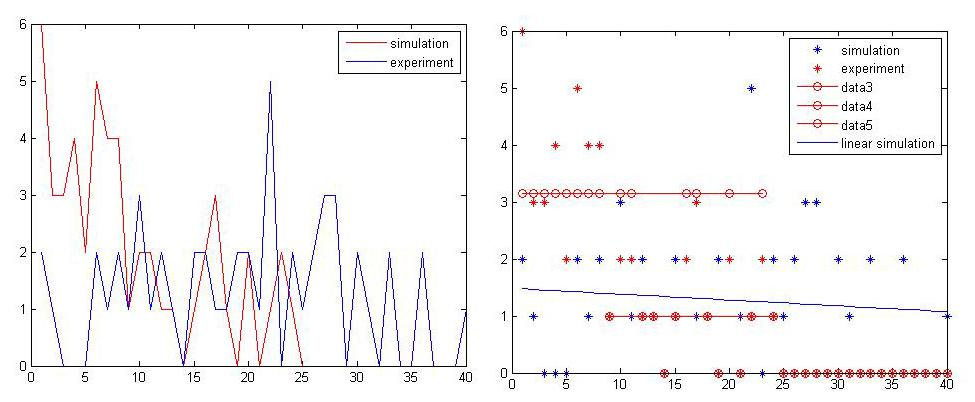Fig.1.11 Simulation about Magnetospirillum Magnetism AMB-1 compared with the experimental group3Experimental group 4 （magnetic field intensit：275mG）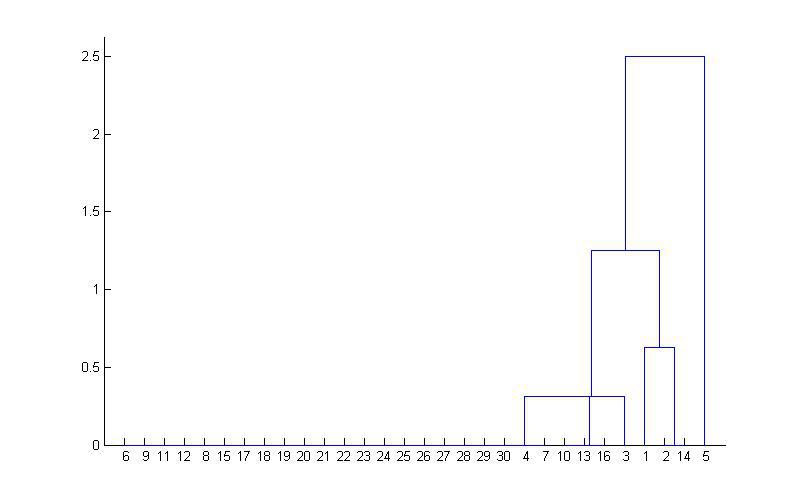Fig.1.12 Clustering results about Magnetospirillum Magnetism AMB-1 experimental group3.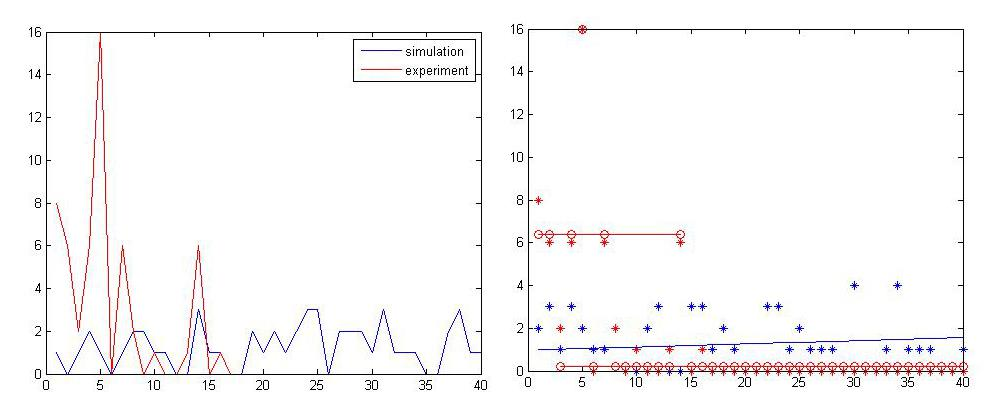Fig.1.13 Simulation about Magnetospirillum Magnetism AMB-1 compared with the experimental group4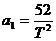According to the results, we can find the relationship between the magnetic field intensity and the dynamic Acceleration. Here is the result of general fitting:
General model Exp1:f(x) = a*exp(b*x)
Coefficients (with 95% confidence bounds):
a = 11.71 (-14.14, 37.57)
b = 0.005176 (-0.004063, 0.01441)
Goodness of fit:
SSE: 75.48
R-square: 0.7333